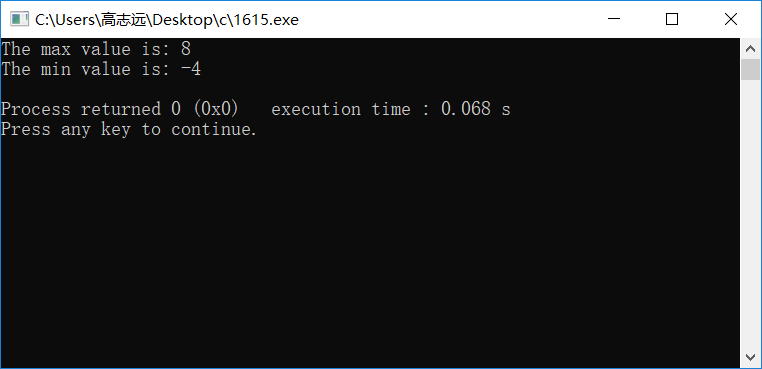# 使用多个return语句

#include <stdio.h>

int findMaxAndMin(int a, int b, int c)
{
int temp = a>b?(temp=a):(temp=b);
int max = temp>c?temp:c;

int temp1 = a<b?(temp1=a):(temp1=b);
int min = temp1<c?(temp1):c;

return max;
return min;
}

int main(void)
{
int a, b, c;
scanf("%d,%d,%d", &a, &b, &c);
printf("The max value is: %d\n", findMaxAndMin(a, b, c));
printf("The min value is: %d\n", findMaxAndMin(a, b, c));
return 0;
}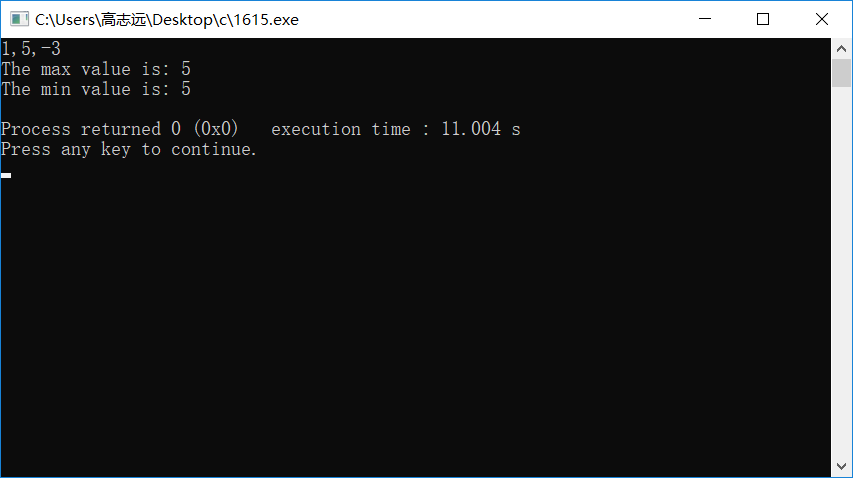#include <stdio.h>

int findMaxAndMin(int a, int b, int c)
{
int temp = a>b?(temp=a):(temp=b);
int max = temp>c?temp:c;

int temp1 = a<b?(temp1=a):(temp1=b);
int min = temp1<c?(temp1):c;

return min;
return max;
}

int main(void)
{
int a, b, c;
scanf("%d,%d,%d", &a, &b, &c);
printf("The max value is: %d\n", findMaxAndMin(a, b, c));
printf("The min value is: %d\n", findMaxAndMin(a, b, c));
return 0;
}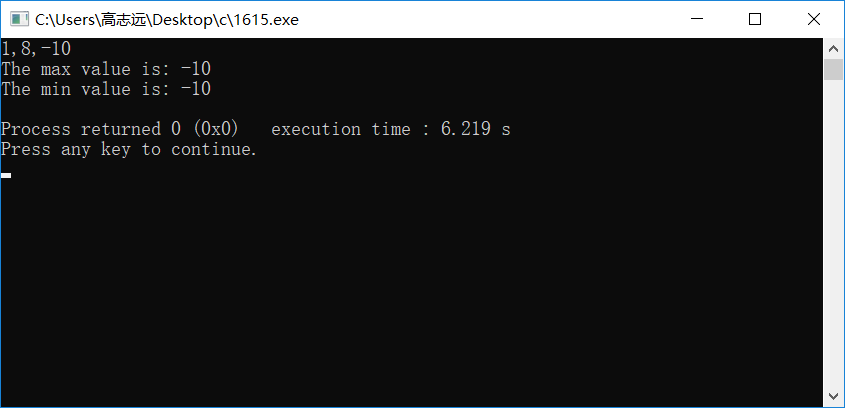# 方法一：使用全局变量

#include <stdio.h>

int max, min;

void findMaxAndMin(int a, int b, int c)
{
int temp = a>b?(temp=a):(temp=b);
max = temp>c?temp:c;

int temp1 = a<b?(temp1=a):(temp1=b);
min = temp1<c?(temp1):c;
}

int main(void)
{
int a, b, c;
scanf("%d,%d,%d", &a, &b, &c);
findMaxAndMin(a, b, c);
printf("The max value is: %d\n", max);
printf("The min value is: %d\n", min);
return 0;
}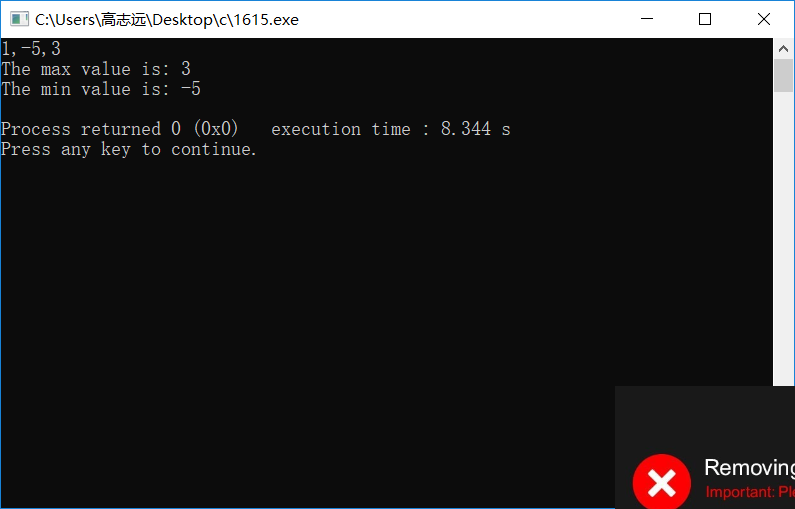# 方法三：利用数组

#include <stdio.h>

void findMaxAndMin(int *pArr, int n)    //传数组给函数需要两个参数（数组名和数组长度）
{
//排序
for (int i = 0; i<n-1; i++)
{
for (int j = 0; j<n-i-1; j++)
{
if (pArr[j]>pArr[j+1])
{
int temp = pArr[j];
pArr[j] = pArr[j+1];
pArr[j+1] = temp;
}
}
}
}

int main(void)
{
int arr;
for (int i = 0; i<3; i++)
{
scanf("%d", &arr[i]);
}
findMaxAndMin(arr, 3);
printf("The max value is: %d\n", arr);
printf("The min value is: %d\n", arr);
return 0;
}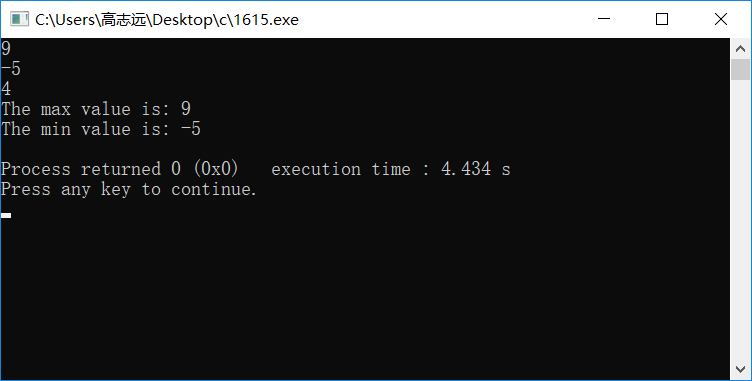Ps：上述排序使用的是冒泡法，使用其他的排序算法也是可以的。

# 方法四：利用结构体

#include <stdio.h>

struct Arr {
int num1, num2, num3;
int min;
int max;
};

void findMaxAndMin(struct Arr *pArr)    //传数组给函数需要两个参数（数组名和数组长度）
{
int t = pArr->num1>pArr->num2?pArr->num1:pArr->num2;
pArr->max = t>pArr->num3?t:pArr->num3;

int t1 = pArr->num1<pArr->num2?pArr->num1:pArr->num2;
pArr->min = t1<pArr->num3?t1:pArr->num3;
}

int main(void)
{
struct Arr arr;
//赋值
arr.num1 = 2;
arr.num2 = -4;
arr.num3 = 8;
findMaxAndMin(&arr);    //传地址到函数
printf("The max value is: %d\n", arr.max);
printf("The min value is: %d\n", arr.min);
return 0;
}